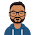### A brief about time in milliseconds and conversion to a human-readable form

Many a time, we come across situations wherein we need to convert the timestamp available(a human-readable form of date-time representation) into milliseconds(long number representation of date-time) or vice-versa.  For example

DateTime in ISO 8601 DateTime in milliseconds
2020-11-15T08:00:00+00:00 1605427200000 ms
2020-07-01T13:00:00+00:00 1593608400000 ms

Seeing the long millisecond number, we generally get confused about how it is converted. We have conversion functions available in almost every programming language which we can think of. But, we always wonder about the logic behind the same. This article will give a brief idea about such questions. Hope you enjoy reading this article. Please share your thoughts in below comment section.

Note: UTC time zone is considered as standard for this article. For other time zones, offset might need to be considered.

## Time in milliseconds

Time in milliseconds is the number of milliseconds elapsed since "1st January'1970 00:00:00.000 UTC". For e.g. 1st January'1970 12:00 PM, value in milliseconds will be 43200000 ms, i.e. 12 hours after 1st January'1970 00:00:00.000 UTC.

12 hours = 12*60*60*1000 ms = 43200000 ms

Table for unit conversions of time.

### Calculations

To convert time in milliseconds, we need to calculate the total milliseconds elapsed since "1st January'1970 00:00:00.000 UTC". Basic formulae to calculate date-time will be:

Time in milliseconds = (((365.24*Y+D)*24*60*60*1000) + (hh*60*60 + mm*60 + ss)*1000 + sss) ms

Where,

Taking 2010-01-10T14:23:59.000+00:00 as reference

Representation of DateTime in ISO 8601 : YYYY-MM-DDThh:mm:ss.sss

YYYY = Year representation. For e.g. 2010

MM = 2-digit representation of month. For e.g. 01(January)

DD =  2-digit representation of Date. For e.g. 10

Y = Year-1970. For eg., if Year is 2010, then the value of Y will be 2010-1970=40.

D= Number of days past in that calendar year. For eg. the date is 10-01-2010, then D= 10, i.e. 10 days are passed in the 2010 calendar year.

hh= 2 digit representation of Hours in 24-hour clock, e.g. 14,

mm=2 digit representation of Minutes, e.g. 23

ss= 2 digit representation of seconds, e.g. 59

sss= milliseconds, e.g. 000

For example, utilizing the above formulae.

2020-11-15T08:23:59.000+00:00

Time in milliseconds = (((365.24*50+320)*24*60*60*1000)+(14*60*60 + 23*60+59)*1000 +000)ms

(18582*86400)*1000 + (50400+1380+59)*1000

1605484800000 + 51839000

1605536639000 ms

We generally see timestamp in ISO 8601 format but if we are provided the time in milliseconds then it is hard to read. We can convert date time information in format like "YYYY-MM-DDThh:mm:ss.sss+00:00" which is nothing but the time format in ISO 8601 format as shown in above example, from given value of time in milliseconds. To do so, let us take "1605622617000 ms" as example.

Let T= 1605622617000 ms

sss= T % 1000 =1605622617000 % 1000 ms = 000 ms

Let tt, represents time in seconds=floor(T/1000 )seconds = 1605622617 s

ss = (tt) % 60 = (1605622617) % 60 =  57

mm = ((tt-ss)/60) % 60 = ((1605622617-57)/60) % 60 = 26760376 % 60 = 16

hh = ((tt-ss-mm*60)/3600) % 24 = (1605622617-960-57)=(1605621600/3600) % 24 = 446006 % 24= 14

Year_d(Year difference) = floor(((tt-hh*3600-mm*60-ss) /86400) /365.24)=floor(((1605622617-50400-960-57)/ 86400) /365.24) = floor((1605571200/86400) /365.24) = floor(18583/365.24) = floor(50.88) = 50

YYYY = 1970 + Year_d = 1970 + 50 = 2020

To calculate month and day values, we need to extract day of the year.

Day_Y_v = (tt - Year_d*365.24*86400 - hh*3600 - mm*60 - ss)/86400 =(1605622617-1577836800-50400-960-57)/86400 = 27734400/86400 =321

Day of the year, Day_Y = ceiling(Day_Y_v)

Note: If the year value is a leap year, then we need to add 1 to the value of day of the year to adjust tropical year and Sidereal year.

Day_Y = ceiling(Day_Y_v) + 1 = (321) + 1 =322

Since 2020 is leap year.

Now the day number 322 is 17th November. Therefore:

DD = 17

MM = 11

Taking the extracted values, date time in ISO 8601 format will be "2020-11-17T14:16:57.000+00:00

1.Another nice article ..thanks !!

1.Thank You :)

2.1.Thank You :)

3.Very well explained 🙂

1.Thank You :)